# Horizontal line

Horizontal line

Do you know what is meant by horizon?

Horizon is a line along which the earth's surface and the sky appear to meet (but they do not actually meet).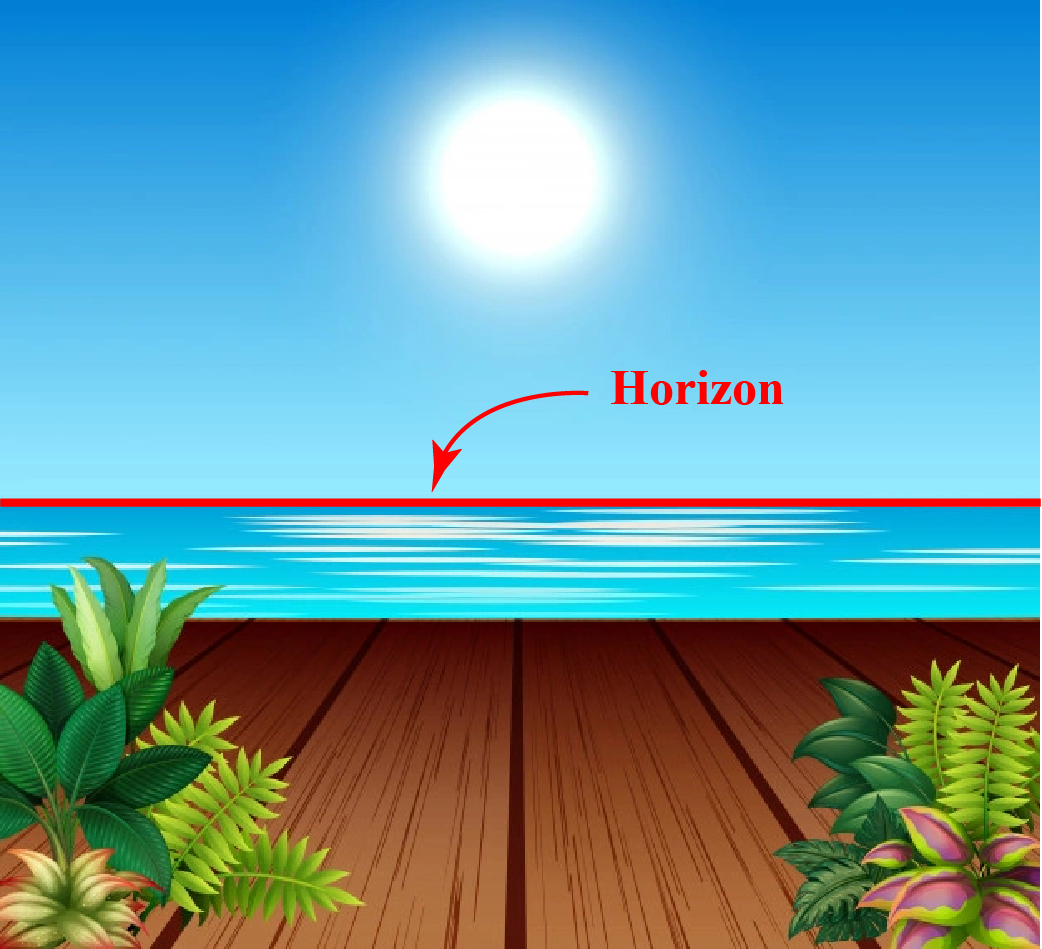The word "horizontal" is derived from the word "horizon".

"Horizontal" means "side-to-side".

Horizontal lines are lines that are parallel to the horizon.

Let's learn in detail about horizontal lines.

## Lesson Plan

 1 What Are Horizontal Lines? 2 Important Notes on Horizontal Lines 3 Solved Examples on Horizontal Lines 4 Interactive Questions on Horizontal Lines 5 Tips and Tricks on Horizontal Lines

## What Are Horizontal Lines?

### Horizontal Line Definition

• In general, horizontal lines are sleeping lines.
• Horizontal lines are lines that are parallel to the horizon.
• Horizontal lines in coordinate geometry are lines that are parallel to the x-axis.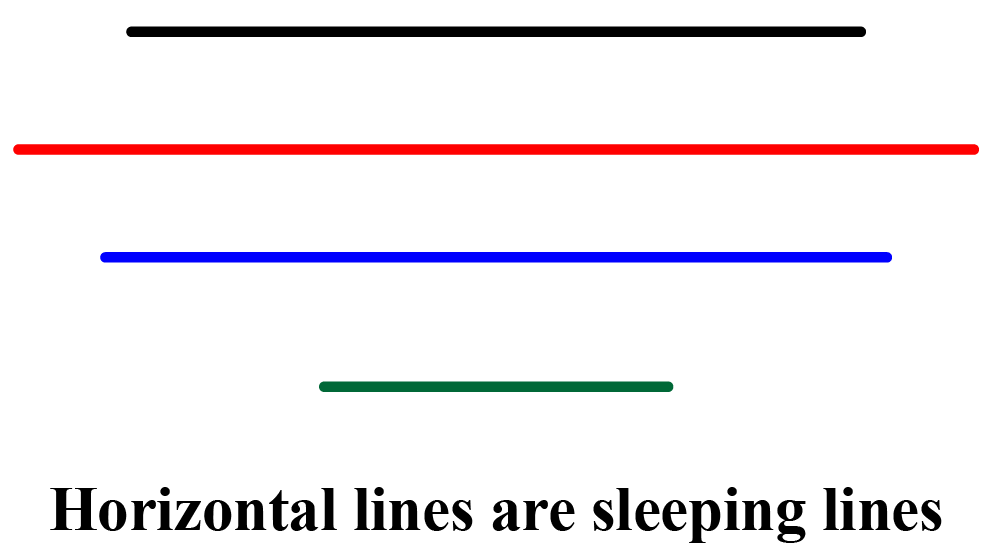## Horizontal Line Images

### Horizontal Line Examples in Real Life

Here are some horizontal line examples in real life.

Other popular examples include steps on the staircase, planks on the railway tracks, etc.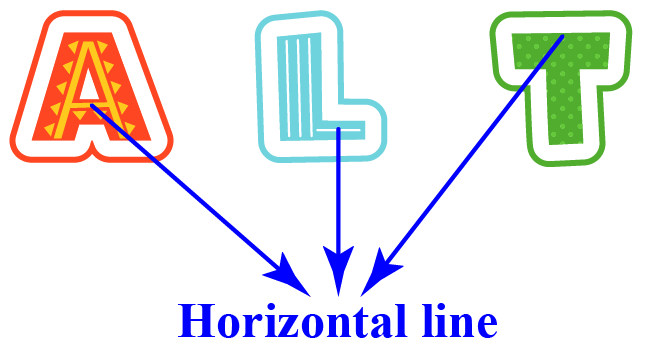### Horizontal Line Examples in Geometry

In geometry, we can find horizontal line segments in many shapes, such as quadrilaterals, 3d shapes, etc.

In coordinate geometry, horizontal lines are lines that are parallel to the x-axis.

Here are some horizontal lines on a coordinate plane.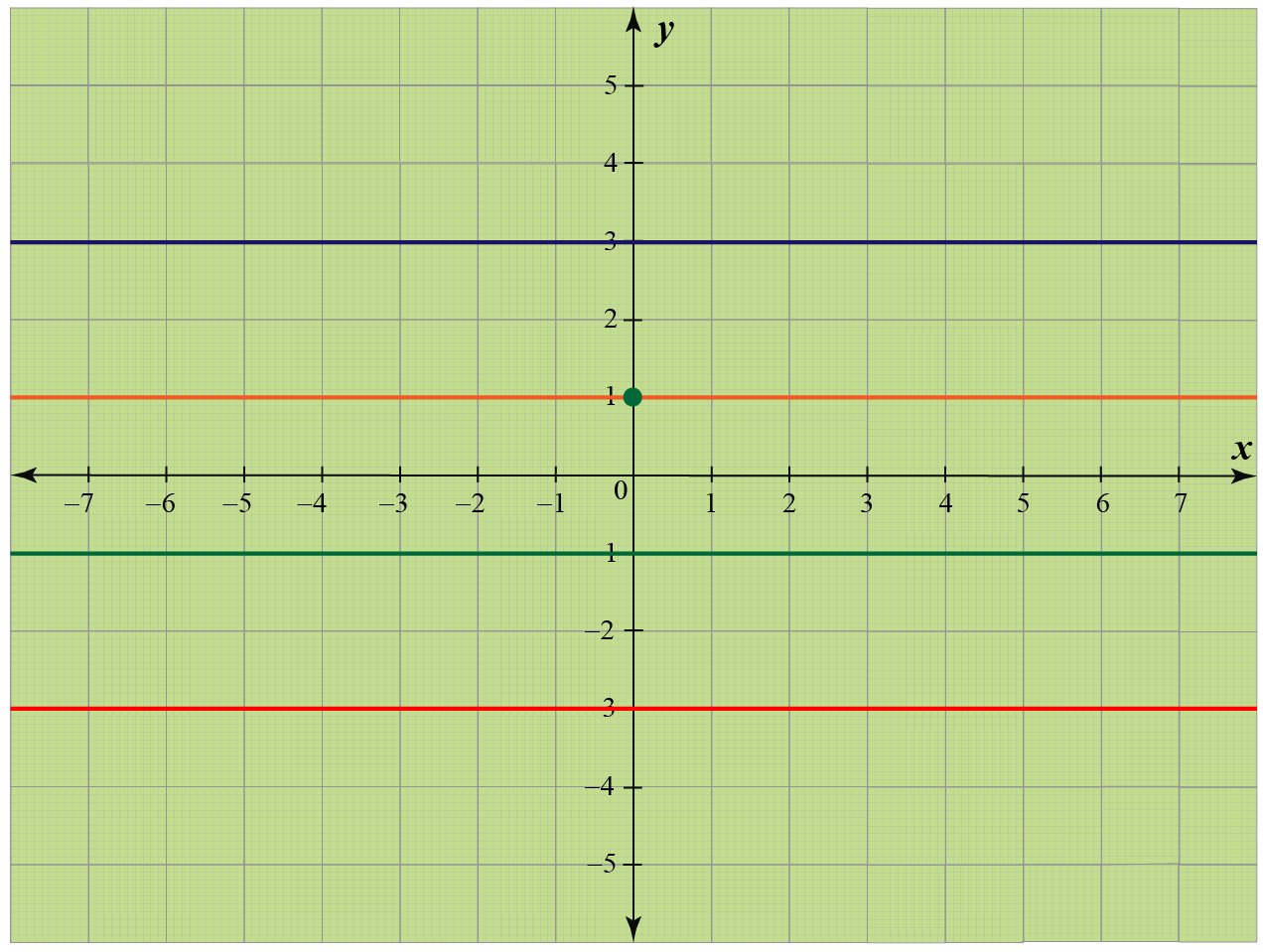## What Are Horizontal and Vertical Lines?

• "Horizontal" means "side-to-side" and a horizontal line is nothing but a sleeping line.
• "Vertical" means "up-to-down" and a vertical line is nothing but a standing line.

"Horizontal" and "vertical" are opposite words of each other.

Horizontal lines and vertical lines are perpendicular to each other.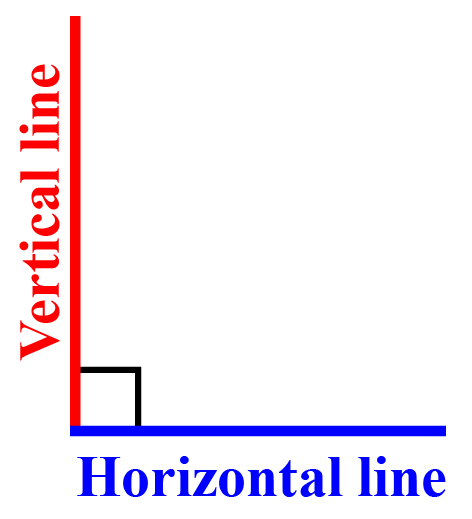## How Do You Make a Horizontal Line?

To make a horizontal line on plain paper, you can use a ruler. Place it parallel to the horizontal edge of the paper and draw a line along the ruler.

But how to draw a horizontal line on a coordinate plane? Let's see.

To draw a horizontal line,

• Place a dot at any random point on the coordinate plane, let's say at (2, -3).
• Identify its y-coordinate. Here the y-coordinate is -3.
• Plot some other points whose y-coordinate is the same as the point plotted. Let's plot (1, -3), (-2, -3), etc.
• Join all the points and extend it on both sides to get the horizontal line.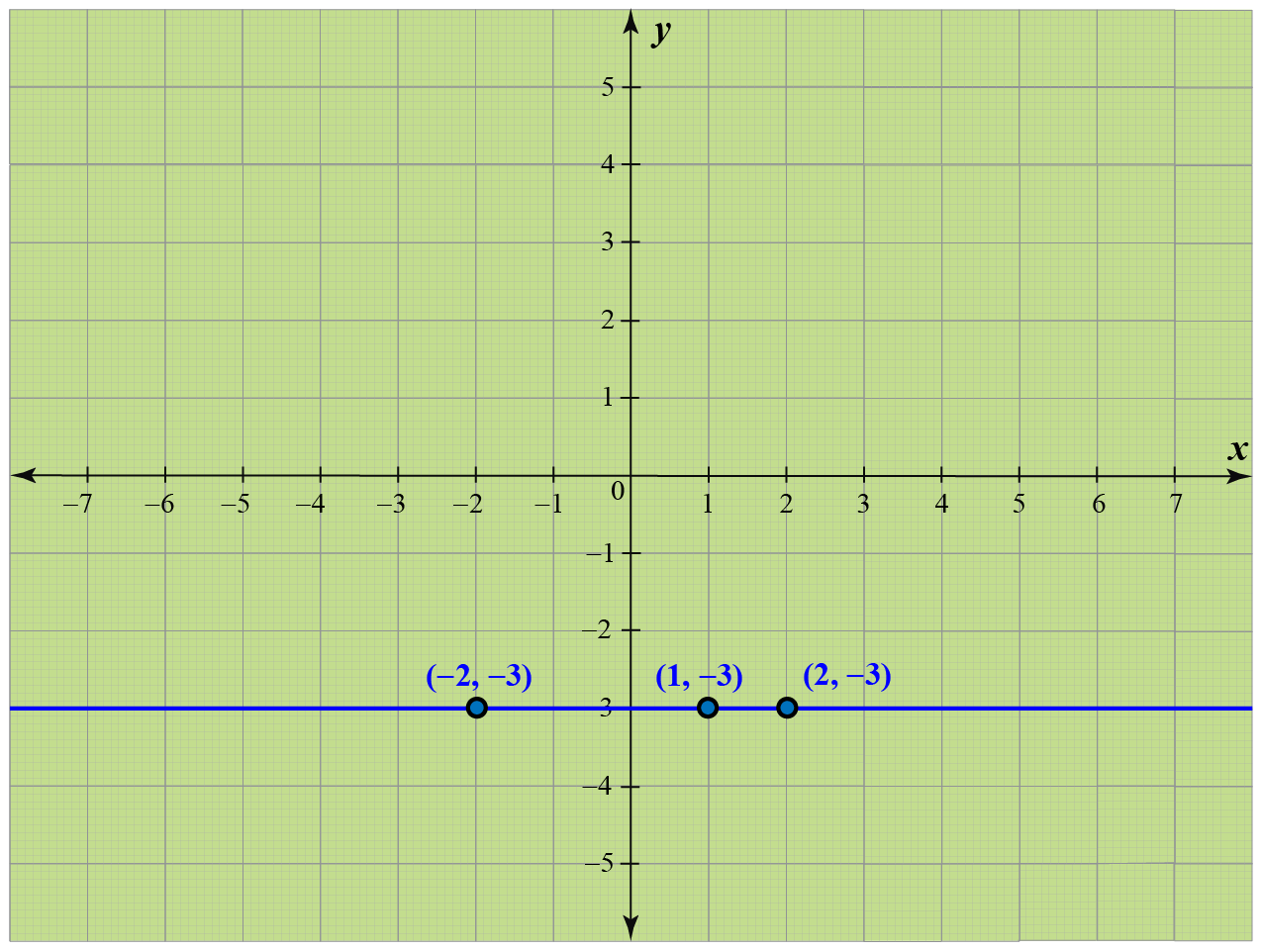## Horizontal Line Equation

In the last section, we can see that the y-coordinates of all the points on a horizontal line are the same.

Thus, the equation of a horizontal line through any point $$(a,b)$$ is of the form: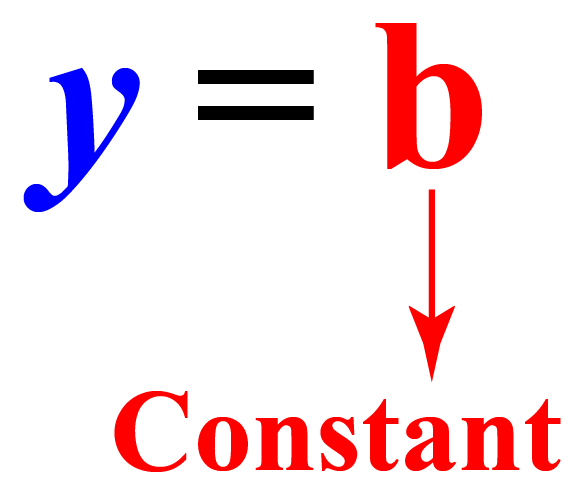Here $$x$$ is absent. It means that the x-coordinate can be anything whereas the y-coordinate of all the points on the line must be $$b$$ only.

The horizontal line slope is 0 as by comparing $$y=b$$ with $$y=mx+b$$, we get the slope to be $$m=0$$.

Here is an example: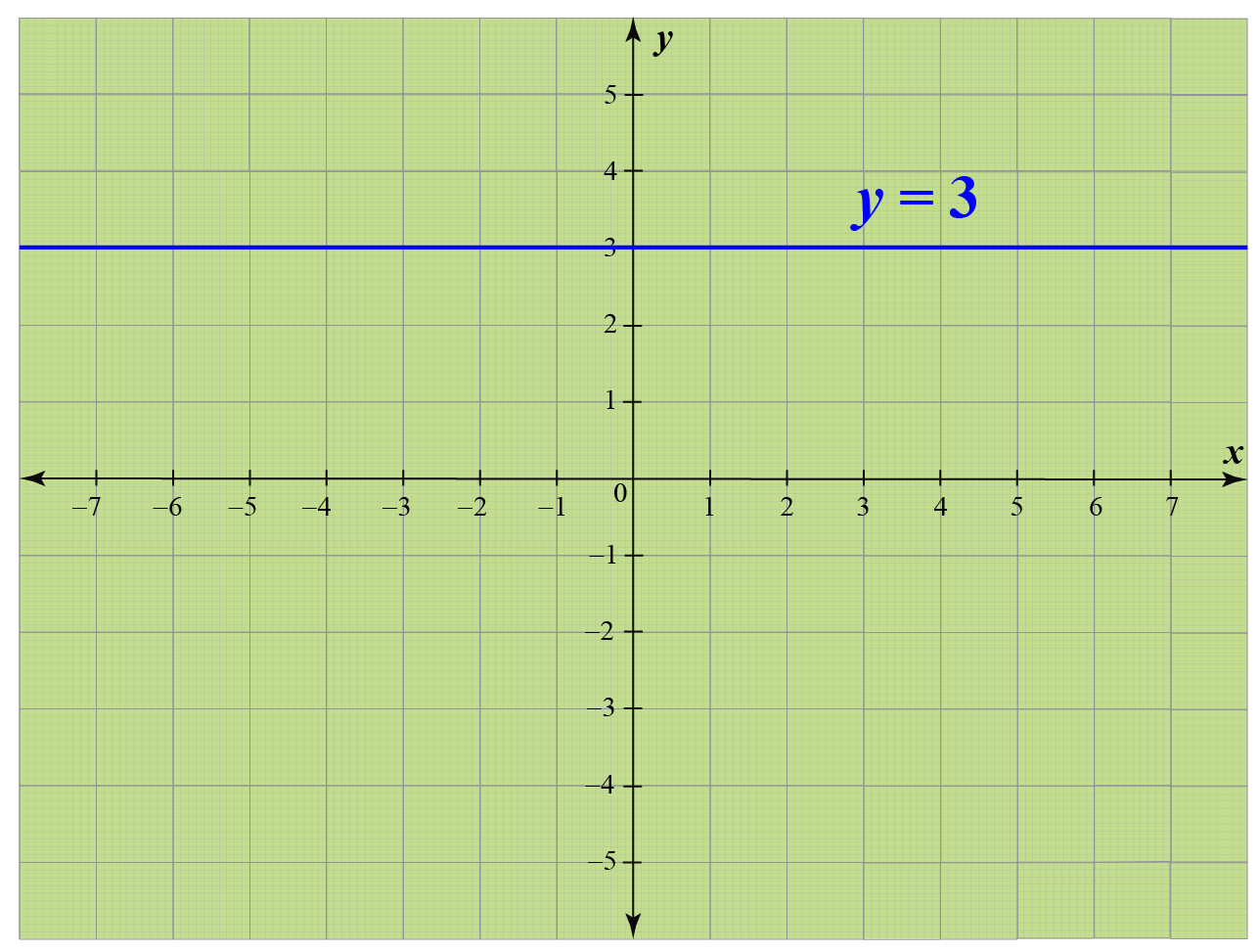Take a look at some of the points on this line: (-5, 3), (-1, 3), (4, 3), (7, 3).

You can see that the y-coordinate of all these points is constant, which is 3. Hence the equation of this line is $$y=3$$.

## How to Use the Horizontal Line?

### Horizontal Line of Symmetry

Horizontal lines are used to represent symmetry.

Horizontal line of symmetry is a horizontal line that makes a shape exactly into two equal parts such that when the shape is folded along that line, one part overlaps the other.

In each of the following figures, the dotted line is a horizontal line of symmetry.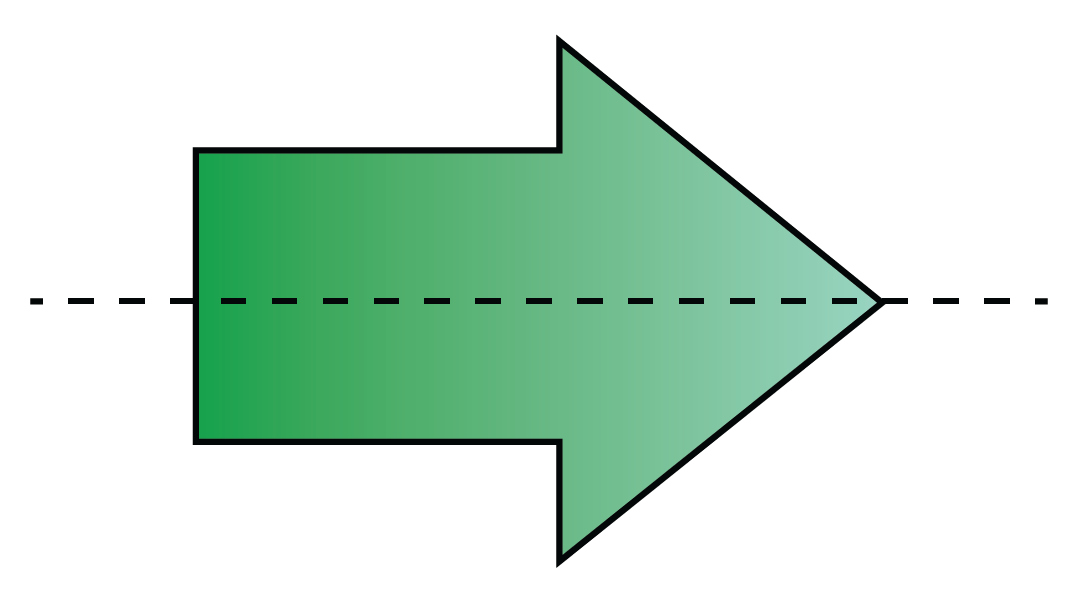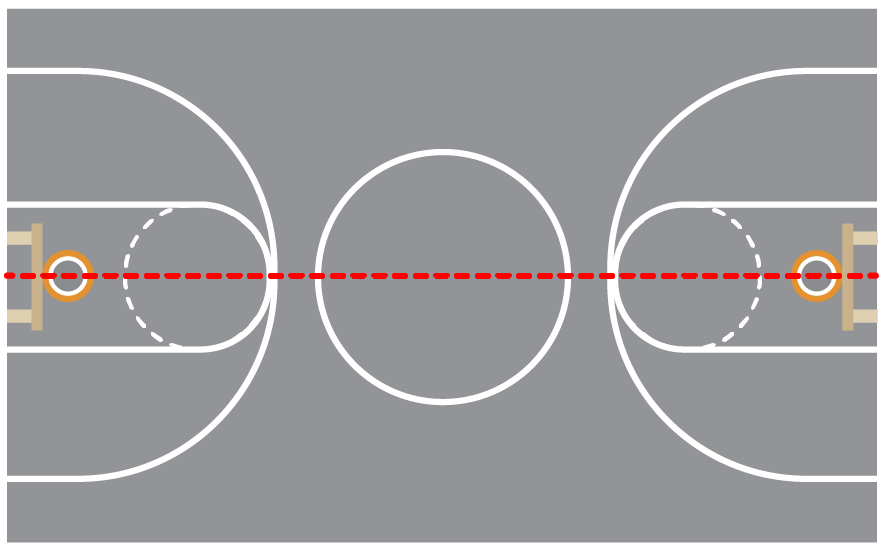### Horizontal Line Test

The horizontal line test is used to determine whether a function is one-one.

According to the horizontal line test, a function is NOT one-one if there exists a horizontal line that passes through more than one point of the graph (of the function).

Example 1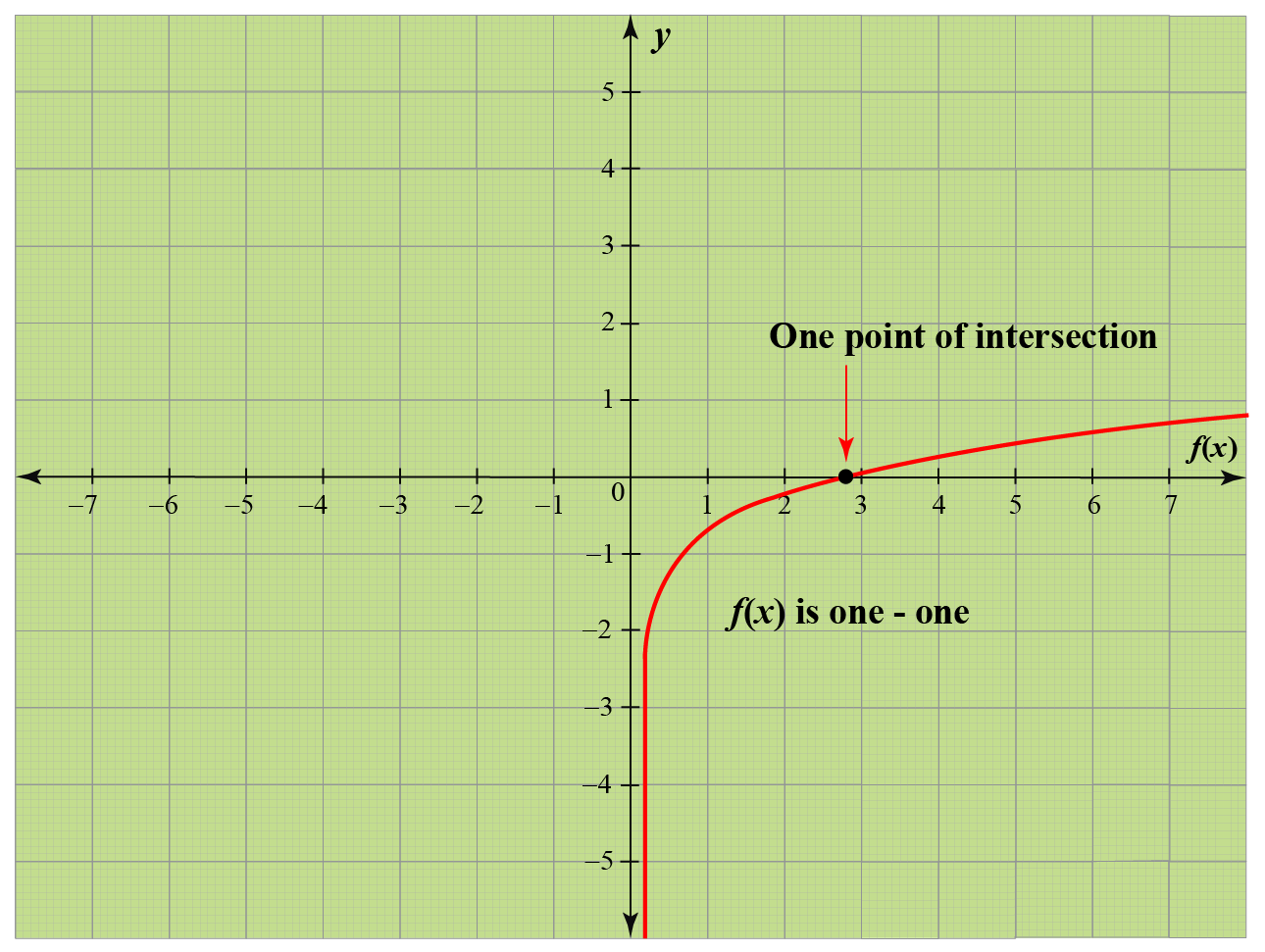Here, $$f(x)$$ is one-one because every horizontal line passes through at most one point of the graph.

Example 2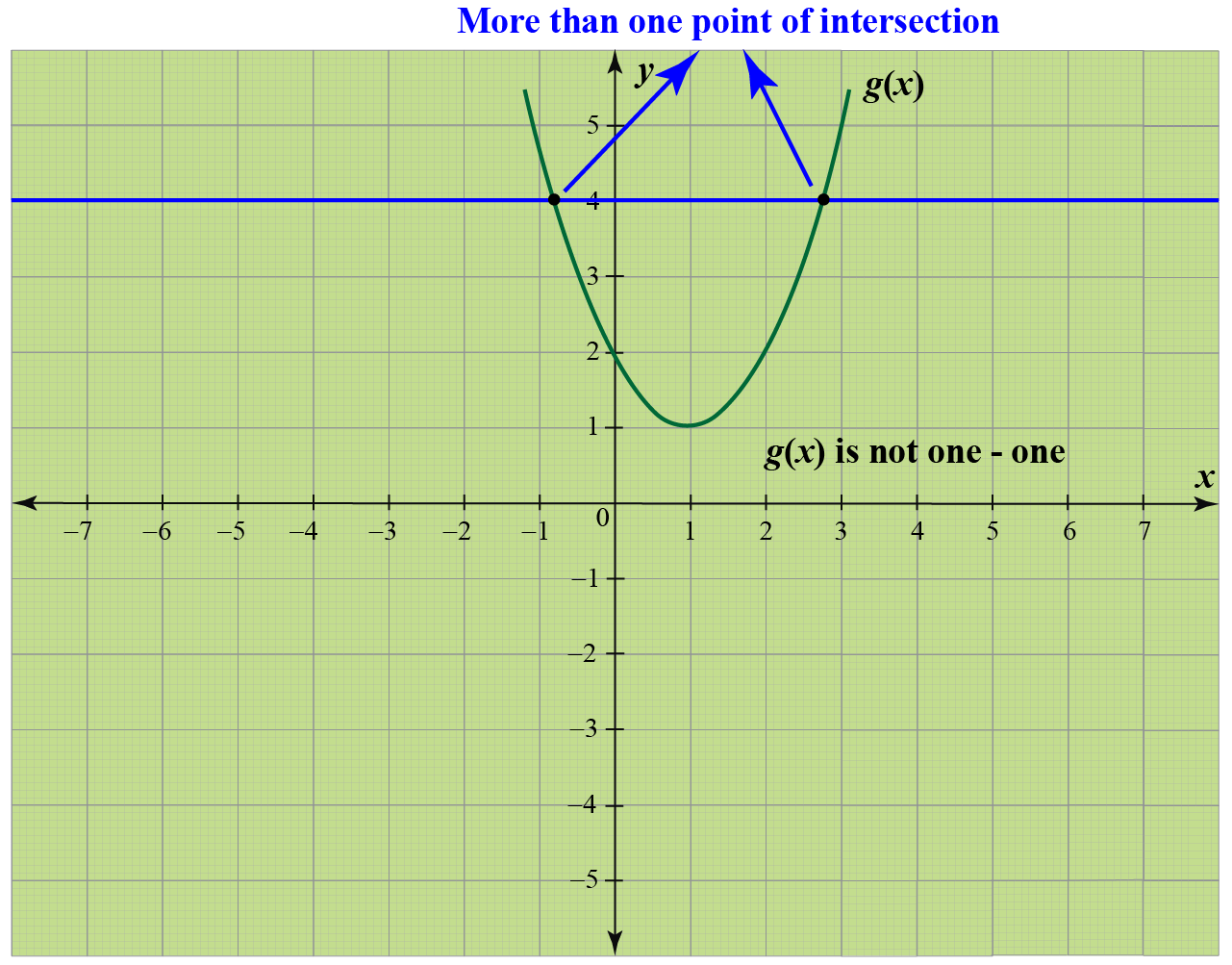Here, $$g(x)$$ is not one-one as there exists a horizontal line that passes through more than one point of the graph.

More Important Topics
Numbers
Algebra
Geometry
Measurement
Money
Data
Trigonometry
Calculus